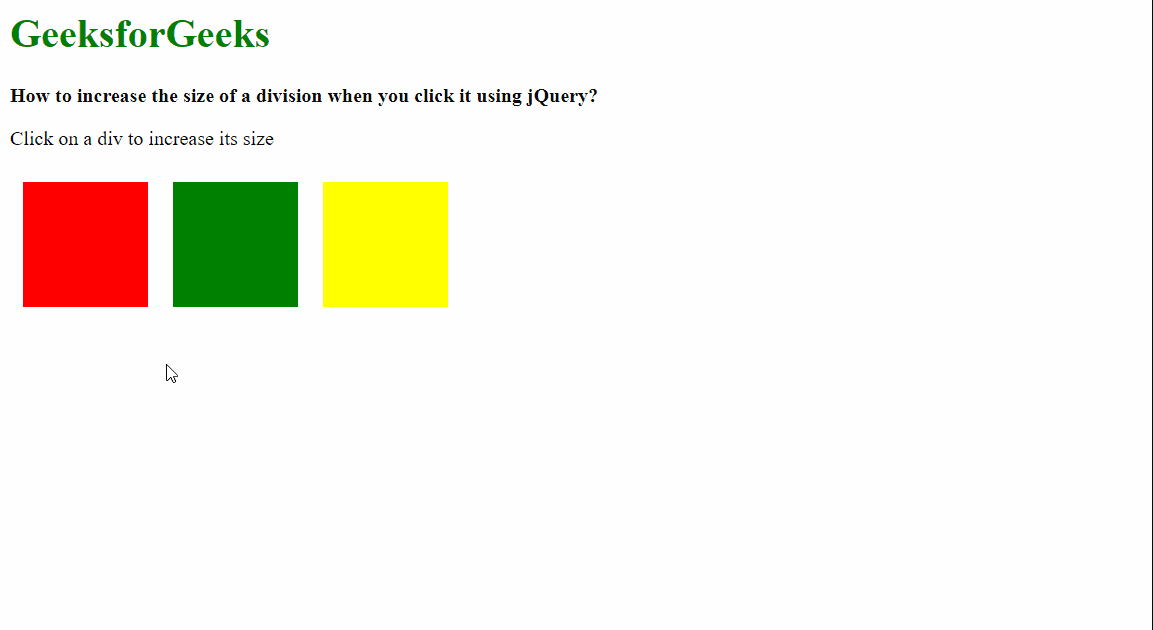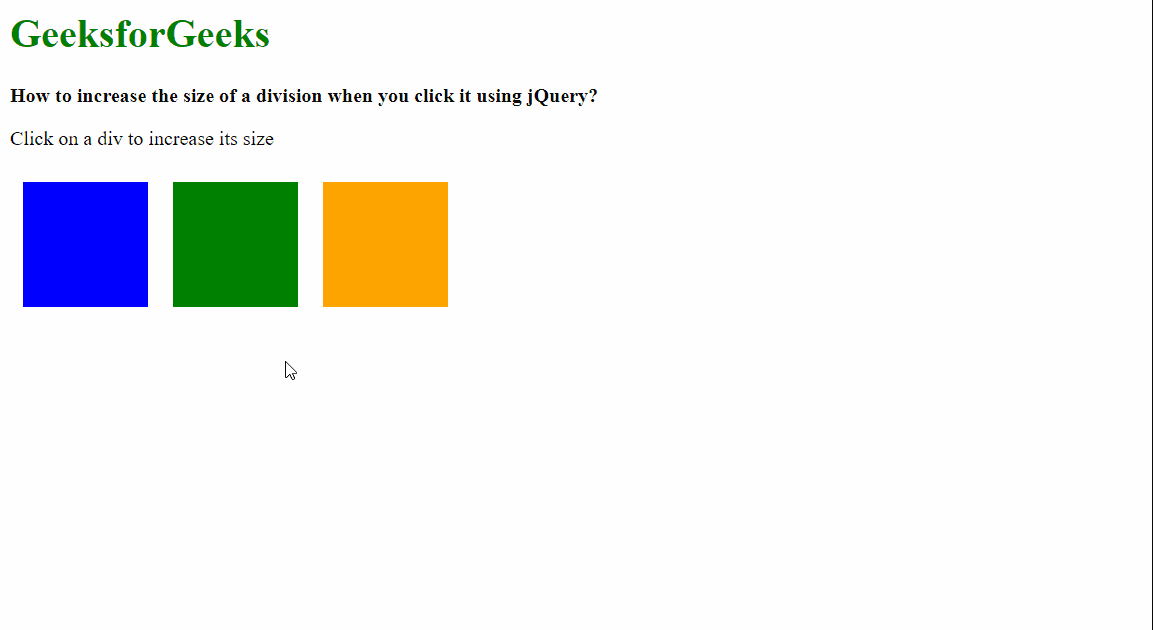Skip to content
Related Articles
How to increase the size of a division when you click it using jQuery ?
• Last Updated : 24 Apr, 2021

In this article, we will learn how to increase the size of a division when you click on it using jQuery.

Approach 1: Using the height() and width() method.

All the divisions on the page are first selected using a common selector and a click binding is applied to trigger the function to increase the size using the click() method. The division that is then currently clicked can then be found out by using this as the selector.

The current height of the element can be found out using the height() method and the current width can be found using the width() method. These are stored temporarily in separate variables. The same methods are then used to set the modified height and width. The new values can be calculated by multiplying the current height and width with a multiplier. This multiplier can be specified as a variable. This will increase the size of the clicked division equally.

We can also use different multipliers to increase the height and width of the divisions separately.

Syntax:

```\$(".box").click(function () {

// Select the clicked element
let curr_elem = \$(this);

// Get the current dimensions
let curr_width = curr_elem.width();
let curr_height = curr_elem.height();

// Set the new dimensions
curr_elem.height(curr_height * increase_modifier);
curr_elem.width(curr_width * increase_modifier);
});```

The below examples illustrate the above approach:

Example:

## HTML

 `<``html``>``<``head``>``  ``<``script` `src``=``"https://code.jquery.com/jquery-3.3.1.min.js"``>``  ````  ``<``style``>``    ``.container {``      ``display: flex;``    ``}`` ` `    ``.box {``      ``height: 100px;``      ``width: 100px;``      ``margin: 10px;``    ``}`` ` `    ``.red-bg {``      ``background-color: red;``    ``}`` ` `    ``.green-bg {``      ``background-color: green;``    ``}`` ` `    ``.yellow-bg {``      ``background-color: yellow;``    ``}``  ``````<``body``>``  ``<``h1` `style``=``"color: green"``>``    ``GeeksforGeeks``  ````  ``<``b``>``    ``How to increase the size of a ``    ``division when you click it using jQuery?``  ````   ` `<``p``>Click on a div to increase its size`` ` ` ` `  ``<``div` `class``=``"container"``>``    ``<``div` `class``=``"box red-bg"``>``    ``<``div` `class``=``"box green-bg"``>``    ``<``div` `class``=``"box yellow-bg"``>``  ```` ` `  ``<``script``>``    ``\$(".box").click(function () {`` ` `      ``// Select the element that``      ``// is clicked on``      ``let curr_elem = \$(this);`` ` `      ``// Set the amount to increase``      ``let increase_modifier = 1.5;`` ` `      ``// Get the current width of the element``      ``let curr_width = curr_elem.width();`` ` `      ``// Get the current height of the element``      ``let curr_height = curr_elem.height();`` ` `      ``// Use the same methods to set``      ``// the new dimensions``      ``curr_elem.height(``        ``curr_height * increase_modifier``      ``);``      ``curr_elem.width(``        ``curr_width * increase_modifier``      ``);``    ``});``  ```````

Output:Approach 2: Using the css() method.

This is similar to the above approach. All the divisions on the page are first selected using a common selector and a click binding is applied to trigger the function to increase the size using the click() method. The division that is then currently clicked can then be found out by using this as the selector.

The current height and width of the element can be found out using by using the css() method and passing the first parameter as “height” and “width“. This will return the current height and width of the division. These are stored temporarily in separate variables after parsing them to an integer using the parseInt() method. The css() method is again used to assign the new height and width bypassing the new value as the second parameter. The new values can be calculated by multiplying the current height and width with a multiplier, similar to the above method.

Syntax:

```\$(".box").click(function () {

// Select the clicked element
let curr_elem = \$(this);

// Get the current dimensions
let curr_width = parseInt(curr_elem.css("width"), 10);
let curr_height = parseInt(curr_elem.css("height"), 10);

// Set the CSS value of the element
curr_elem.css({

// Set the new height and width
width: curr_width * increase_modifier,
height: curr_height * increase_modifier,
});
});```

Example:

## HTML

 `<``html``>``<``head``>``  ``<``script` `src``=``"https://code.jquery.com/jquery-3.3.1.min.js"``>``  ````  ``<``style``>``    ``.container {``      ``display: flex;``    ``}`` ` `    ``.box {``      ``height: 100px;``      ``width: 100px;``      ``margin: 10px;``    ``}`` ` `    ``.blue-bg {``      ``background-color: blue;``    ``}`` ` `    ``.green-bg {``      ``background-color: green;``    ``}`` ` `    ``.orange-bg {``      ``background-color: orange;``    ``}``  `````` ` `<``body``>``  ``<``h1` `style``=``"color: green"``>``    ``GeeksforGeeks``  ````  ``<``b``>``    ``How to increase the size of a ``    ``division when you click it using jQuery?``  ````  ``<``p``>Click on a div to increase its size`` ` ` ` `  ``<``div` `class``=``"container"``>``    ``<``div` `class``=``"box blue-bg"``>``    ``<``div` `class``=``"box green-bg"``>``    ``<``div` `class``=``"box orange-bg"``>``  ```` ` `  ``<``script``>``    ``\$(".box").click(function () {`` ` `      ``// Select the element that``      ``// is clicked on``      ``let curr_elem = \$(this);`` ` `      ``// Set the amount to increase``      ``let increase_modifier = 1.5;`` ` `      ``// Get the current width of the element``      ``// and parse the value to integer``      ``let curr_width = ``          ``parseInt(curr_elem.css("width"), 10);`` ` `      ``// Get the current height of the element``      ``// and parse the value to integer``      ``let curr_height = ``          ``parseInt(curr_elem.css("height"), 10);`` ` `      ``// Set the CSS value of the element``      ``curr_elem.css({`` ` `        ``// Set the new height and width``        ``// after multiplying``        ``width: curr_width * increase_modifier,``        ``height: curr_height * increase_modifier,``      ``});``    ``});``  ```````

Output:My Personal Notes arrow_drop_up